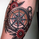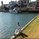# Volume Accumulation Percentage Indicator [LazyBear]

41652 views
Volume Accumulation Percentage Indicator (VAPI) is a variation of the classic volume-accumulation indicators. It uses volume the same way as OBV except that it assigns volume weights based on intraday volatility .

In a positive trend, if the stock price is close to a (local) new high, the VAPI should be at its maximum as well (and vice versa for a negative trend). If the market is looking for a direction, if the price is in the bottom side of his current trading range and if, at the same moment the VAPI osc slopes up, there is accumulation on the stock and that the prices should start to climb. The SELL signal is given when the VAPI decreases (distribution). Divergences are usually very effective too.

Tuning the "length" parameter may be needed for your instrument (default is 10), do let me know if you find a different value to be optimal for majority of instruments.

List of my public indicators: http://bit.ly/1LQaPK8
List of my app-store indicators: http://blog.tradingview.com/?p=970
```//
// @author LazyBear
// List of my public indicators: http://bit.ly/1LQaPK8
// List of my app-store indicators: http://blog.tradingview.com/?p=970
//
study("Volume Accumulation Percentage Indicator [LazyBear]", shorttitle="VAPI_LB", overlay=false)
length=input(10, title="Time Periods")
x=(2*close-high-low)/(high-low)
tva=sum(volume*x,length)
tv=sum(volume,length)
va=100*tva/tv
zl=plot(0, color=gray, title="ZeroLine")
vac=gray
val=plot(va, color=vac, style=histogram, title="VAPI Histogram")
us=va<0?0:(va==0?va  :va), ls=va>0?0:(va==0?va:va)
ux=plot(us, color=gray, style=circles, title="DummyU"), lx=plot(ls, color=gray, style=circles, title="DummyL")
fill(ux,val,color=red, transp=50, title="NegativeFill"), fill(lx,val,color=lime, transp=50, title="PositiveFill")
plot(va, color=gray, linewidth=2, title="VAPI Osc")```
List of my free indicators: http://bit.ly/1LQaPK8
List of my indicators at Appstore: http://blog.tradingview.com/?p=970reminds me a bit of a squeez indcator you made, you basically changed the way i now think on the market, you and John carter have changed my p/l ths year, really like what you are doing here keep up the great work.

much respect !